#### meshquality.f

In this routine the quality of each element is determined. To this end the ratio of the largest edge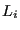to the radius of the inscribed sphere is used. One can prove that the radius of the inscribed sphere of a linear tetrahedral is three times the volume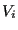divided by the sum of the area of its faces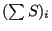. Therefore, the quality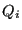for element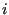can be written as: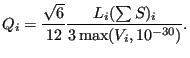(715)

The factor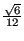is such that the quality of an equilateral tetrahedron is 1. For all other tetrahedra it exceeds 1. The larger the value, the worse the element. The cut-off of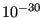was introduced to avoid dividing by zero or getting a negative value.